American Journal of Aerospace Engineering
Volume 2, Issue 3, June 2015, Pages: 11-29

On the Problem of Neutrino Mass in the Aspect of the Detected Spatial Anisotropy of Temporal Variations of Solar Neutrinos Flux

A. G. Syromyatnikov

St. Petersburg University, Department of Physics, Universitetskaya Nab., St. Petersburg, Russia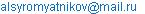A. G. Syromyatnikov. On the Problem of Neutrino Mass in the Aspect of the Detected Spatial Anisotropy of Temporal Variations of Solar Neutrinos Flux.American Journal of Aerospace Engineering.Vol.2, No. 3, 2015, pp. 11-29. doi: 10.11648/j.ajae.20150203.11

Abstract: Mass of the muon and tau-neutrinos are calculated. Set the integer 2:5 ratio between the mass of muon neutrinos and muon ultra-light weight torsion. Found that the calculated mass of the tau-neutrino is the same as the largest with heat 3/2 T relic neutrinos. In the standard model T = 2K. Considering the temperature of the relic neutrinos as the effective gravitational temperature TΓ = 2/9 M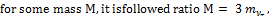corresponding to reactions of the dissolution of the relic neutrinos by three sterile active Tau-neutrinos. Solar neutrino flux measurements data and its temporal variations in the Chlorine-Argon experiment for the period 1970-1994, examined with particular attention near dated frontiers of solar activity (FSA), which defined by means of the key criterion of 2ln2 in point a significant manifestation of the influence the Sun eccentric planets. Review shall be carried out within the framework of the standard model, taking into account the gravity. The results are presented in the form of pie charts in a consecutive temporary scan on a direct ascent of Earth's orbital position α, recalculated according to the date of the middle of each of the series of measurements. The corners of the sectors 35° and more defined for temporary length of each dimension.The amplitude of the sector is equal to the measured magnitude of neutrino flow minus the constant component 2.57 SNU. All charts show the priority direction of the solar neutrino flux detector checked by angles α = 271°, δ = 40º (-15º) axis of anisotropy of Galactic Gamma-radiation, as well as a number of less significant in transverse to the axis of the direction. Researched the relationship between solar activity, seismic activity of the Earth and neutrinos flows registered in chlorine-argon experiment for the period 1970-1994, as well as in the SAGE for the period 1970-2008years.There are correlations of solar activity with seismic activity of the Earth and the positive correlation between seismic activity of the Earth and the neutrino flow variations, reaching 95% when taking into account the peculiarities of the matching of dates of solar maximums with FSA dates 1978, 1993-5 and 2002.It turned out that all known and perhaps predicted on 2018 year the greatest average annual values of the flow of solar cosmic rays which defines corresponding dose fall exactly on the years of FSA defined on the planets eccentric.

Keywords: Solar Activity, the Seismic Activity of the Earth, Neutrino Flux Registrated in Chlorine-Argon Experiment and Sage, Anisotropy, Correlation

1. Introduction

Currently we have only experimental lower bounds for neutrinos and practically do not have significant theoretical guidance for its difference from zero . However, first, without differences of mass neutrinos from zero was impossible explanation of lack in comparison with calculations based on the standard solar model solar neutrino flow has been measuring in chlorine-argon experiment , which for about 24 years held in the mines in Homestake, United States, as well as gallium-germanium experiment SAGE  neutrino Baksan Observatory of NRI (RAS), conducted from June 1990 to 2005 years, if the mass of the electron neutrino is anywhere from 10-4-10-8 eV. Secondly, considerable time variation found neutrino flux, which also does not conform to the standard solar model . There is a negative correlation of solar activity in Wolf numbers on the number of sunspots with seismicity of the Earth and the positive correlation between seismic activity of the Earth and variations of neutrino flow . Long before that, at least in  3453 outbreaks points > 2.2 f It was determined the direction of anisotropy of distribution of sunspots in the field standard Apex Sun at the second equatorial coordinate system: α = 277° ± 5°, δ = +29º ± 5°; in the same direction is a maximum of cometary α = 271°, etc. In [6-7] presents the results of theoretical and experimental studies of space-time anisotropy angular distribution of Galactic Gamma-radiation in frame of the generation mechanism of cosmic rays by the method of direct conversion of extragalactic gamma-rays to the current on spin shock waves. It is shown that the angular axis anisotropy of Galactic Gamma-radiation has the following coordinates: Galactic longitude l = 96°, right ascension α = 271°. There is the main maximum in this direction on a chart of the average annual release of seismic energy on among the strongest earthquakes that have occurred in the period 1990-2015 years (according to the Internet site: ceme.gsras.ru).Data of earthquakes on the CEME GS RAS base averaged sliding window method on 5 points. Then was done subtraction of geo-background. Then the resulting data were divided into 14° sectors. Without these operations with a data source on the chart does not receive the anisotropy. Generation of spin shock waves in the Sun is unquestionable, because the magnetic field jumps recorded since the beginning of regular observations. Their detection in the Sun means the isotropy around solar space-time according to theorem Neuter: conservation of spin angular momentum as the law of dynamics of spin shock waves means the isotropy of space-time.

The main objective of this work will be to identify and define the axes of anisotropy of flux variations of solar neutrinos.

The most interesting  possible explanation of anticorrelations neutrino flux with solar activity, confirmed the same detector in 1990 year  it was suggested by M. B. Voloshin, M. I. Vysotsky and L. B. Okhun (Russian Nuclear physics, 1986, vol. 44, pp. 440, 544, 546). According to their hypothesis, the electronic neutrino with magnetic moment 10-11 Bohr’s magneton as a result of the influence of the solar magnetic field becomes sterile and does not interact with the nucleus of chlorine. Maximum sterilization neutrino occurs during peak solar activity (1978 year – S. A. G.), when the magnetic field of the Sun is the greatest. In theory a sterile neutrino below we follow works [8-10]. In the range 1-200 GeV mass sterile neutrinos, shared with the particles of the standard model, You can be guided by their linear relationship with the masses of the chemical elements [11-12]. In particular, this limit on the mass of the neutrino in the order of 0.3 eV ·c-2, that corresponds to modern top mass measurements of electron neutrinos 2.2 eV . On the other hand, their detection in the planned experiments on the LH Collider  can give additional information about the linear law mass particle/atomic weight.

2. Mass of Neutrinos

According to GUT (see  p. 35) the left light neutrinos mass is determined by the ratio of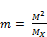,

where m is the mass of spinors-quarks or leptons, and МХ = 1014 mp -scale masses, GUT mp is the mass of a proton.

This gives m = 0.28 eV ·c-2 on the t-quark mass 173 GeV ·c-2. On the mechanism of "see-saw" the mass of neutrinos is proportional to the square of the fermion masses of the same family. It is expected (see.  p. 35) that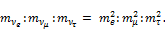Fixing M as the mass of muon 105.66 MeV ·c-2  on the formula for the mass of the muon neutrinos get value 2.5874 mT = (5/2 + 0.035) mT, where mT = 4.70·10-8 eV- axial torsion weight , freezing in heavy electrons spin density distribution. This is an integer value with a precision 3.5% complete (the law of conservation of energy in the center of mass) in the reactions of γ/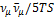of muon neutrino pair production by photon with followed the release of the five lightest axial torsions TS. Of course, in case of a non-zero neutrino pulse more likely could create from one torsion.

Fixing M as the tauon mass 1777 GeV ·c-2 , is similar to the formula for the mass of tauon neutrinos we get the value of 0.000301 eV ·c-2. The estimated mass M of the tau neutrino is the same as the largest with heat energy 3 /2 T of relic neutrinos with a spread of 14%. In the standard model T around 2K. However, considering the temperature of the relic neutrinos as the effective gravitational temperature TΓ = 2/9 M for some M, mass of m = 3/2 TΓ ratio turns out M = 3 m, corresponding reactions of the dissolution of the relic neutrinos by three sterile active Tau-neutrinos . On the theory  in this energy range can only be one sterile neutrinos. A life time of sterile neutrinos, such a mass of not less than the lifetime of the proton. On the other hand this value  evaluation of the upper limit of the mass of the tau neutrino 10 MeV reaches 13 billion years. When considering the effective gravitational temperature commonly used generalized principle of equivalence: the system of graviting fermions, which fit this definition is equivalent to the quantum state oscillator in the coordinate system, moving with some acceleration. When the effective temperature of the oscillator is proportional to acceleration. When the effective temperature of the oscillator is proportional to acceleration. Thus, we see that mass of relic neutrinos, tau-sterile neutrinos generated as dark matter [8-10], in some ways generated by accelerating expanding universe. More accurate staging of this connection is given in .

To conduct the experiment on finding heavy axial torsion with weight 3 TeV at the LH Collider of interest reaction γ/Z/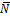N/TS. From kinematics, it follows that the mass of the sterile neutrino N must be in the order of 1.5 Tev. Conversely, when the mass of sterile neutrinos at least 1.5 Tev mass heavy axial torsion shall be not less than 3 TeV. Therefore, accounting of loops of sterile neutrinos can lead to substantial growth of the axial torsion weight.

3. Correlation of Neutrino Fluxes from Seismicity of the Earth

In the experiments (see  reference ), conducted in 2009-2012 years simultaneously in Moscow at the Institute of terrestrial magnetism, ionosphere and radio propagation RAS (TMIRAS) and at the point of integrated observations of the Kamchatka branch of geophysical survey of RAS near Petropavlovsk-Kamchatsky were registered neutron flows associated with earthquake with a magnitude M 8.8 in Chile February 27, 2010 year, volcanic eruption in Iceland, March-April 2010 years, an earthquake with a magnitude M 9 March 11, 2011 in Japan, the earthquake with a magnitude M 8.6 in Indonesia April 11, 2012. On complex for registration of neutrons used gas-discharge counters filled with gas helium-3.These devices detect thermal neutrons, the energy which is 0.025 eV and fast neutrons with energy greater than 2 MeV. In these events increase the particle flows began to occur a few months before these events. When this happened as a continuous monotonic increase in the flow of particles and individual short-term increase with amplitude on the minute data from several thousand, hundreds of thousands of per cent. The duration of these spikes ranging from several minutes to several hours . These and other data displayed in Figure 1.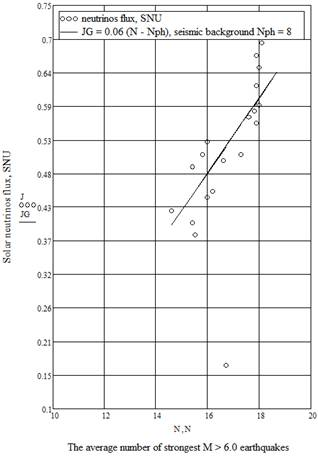Figure 1. The flow of solar neutrinos for the 1970-1994 year s depending on the release of seismic energy on average among the strongest root from 400 km of earthquakes (, fig. 3, p. 8). Point at the bottom is dated 1979 year of solar maximum, which fell on the frontier of solar activity (FSA) 1978 year . Neutrino highs everywhere for 45 years of observations fall on the dates of the FSA. Here, at the time of solar maximum, the negative correlation is replaced by a positive. Therefore, this point falls from JG linear dependence graph.

Shown in Fig. 1 data is approximated by a linear dependence between the flow of solar neutrinos and the release of seismic energy on the average annual number of the strongest deep from 400 km earthquakes .Point at the bottom is dated by the 1979 year of the Frontier of solar activity (FSA) 1978 . Neutrino maximums mainly occur in periods of recession of solar activity (see, e.g., ), as well as 45 years observing everywhere for fall on dates FSA. Here, however, at the time of solar maximum, the negative correlation is replaced by a positive. Therefore, this point falls out of graphics JG of the linear dependence. As a result, after removal of this sample point correlation coefficient of the neutrino fluxes from seismicity of the Earth grows dramatically from 73% to 95%.Standard deviation of data from linear dependence JG 0.03 ± 0.05.Relative variation is within 7%.

These data demonstrate a deep solar-terrestrial relationships. In the author's treatment by the method of [17,18] stochastic seismic processes occur with 22.3 years period the solar cycle and inside the loop reaches a peak dates FSA, caused by the orbital motion of the Sun due to the planetary eccentric. Telling in this regard, parade of planets from the date FSA 1986 etc., when all the planets line up so that the center of mass of the solar system (CMSS) goes beyond the solar disk. The interval between two FSA inside the solar-terrestrial 22-year cycle for 1000 years is an average of 8 years. The offset maximum dates the strongest earthquakes regarding the dates for FSA 200 years does not exceed 2 days. On the other hand CMSS output beyond the solar disk on the date the FSA1978, 1986, 1993-4, 2002 and may 2018 years. In theory [14,15] at the time of the achievement of the FSA has an imbalance of the gravyelectroweak protective potential, which is proportional to Newtonian gravitation potential, inside (220 MeV) and out of the Sun. This happens, as shown above, mostly away from solar maximum to the number of Wolf, so the formation of sunspots and the output of the neutrinos are going mainly in antiphase.So perhaps sunspots processes generally stem from some other, more energy processes.

Experiments on the LH Collider reached a threshold energy 3 TeV differences between gravitational and inertial masses of GUT scales. Physical effects of this estimated range  on the protons from the Sun at the scale of the solar spots size of 20-50th. km 700 km deep photosphere is sufficient to ensure the increased solar activity for billions of years.

Anyway, identified correlations neutrino fluxes from deep earthquakes approach  can be generalized to the entire seismic land. The results are shown below in Fig. 2.

Shown in Fig. 2 data as well as in Fig. 1 are approximated by the linear dependence between the flow of solar neutrinos and the release of seismic energy on average among the strongest earthquakes m > 6.6 according to the earthquake catalog GS RAS CEME  for 1990-2008 years in averaged over five points. The standard deviation of the data from the linear dependence JG on neutrino- 0.06 ± 9.8 SNU, among the strongest earthquakes 0.06 ± 2.8.Relative variance for neutrinos is within 10%. This is somewhat more than for deep earthquakes, but takes into account all the points.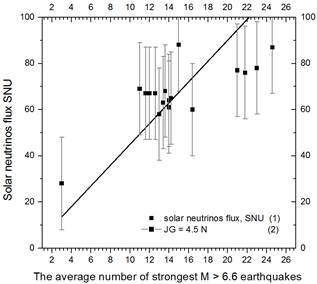Figure 2. The flow of solar neutrinos for 1990-2008 years according to (, fig. 2, p. 4) averaged over three points depending on the release of seismic energy on average among the strongest M > 6.6 earthquakes catalogue CEME GS RAS  averaged over five points.

4. Correlation of Solar Activity on the Annual Course of the Earthquakes

Frontiers of solar activity above under paragraph 2 determined by the time the center of mass of the solar system beyond the solar disk (according to the results of the calculations of the main Astronomical Observatory RAS officer G. Ya. Vasilyeva, A. A. Shpital′naya and others, the movement of the Sun relative to the center of mass of the solar system (see, e.g., ). The period between the two closest Frontiers is within 22 year solar cycle, with maximums of solar activity may not match, very variable and the average is about 8 years old. Near the Frontiers of the solar system as a whole is destabilized (it is a correction of its Galactic orbit) -disturbed balance between the protective gravielectroweak energy potential (PGEWP)  of the center of mass of the solar system and Galactic Centre on the one hand, and synergistic gravitational partially screened potential Universe [14,16]. This is accompanied by powerful emissions of substances and radiation of the Sun, as well as bursts of seismic activity of the Earth, the following followed by transition through Frontiers delayed by 2 days on average for the period of regular observations from beginning of the 16th century.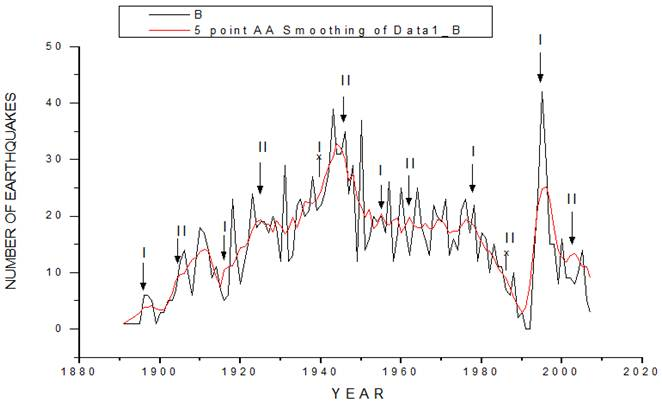Figure 3. The annual course of the strongest earthquakes on the frontiers of solar activity (FSA). The date of the first (I) and second (II) FSA marked by arrows. Shows the smoothed curve by the sliding window method with averaging on 5 points (the smoothed curve by 5 points).Two frontiers 1940 and 1986, inconspicuous amid rapid growth/decline of seismicity, indicated by an arrow with the icon "x".

Here is a comparison of solar output at Frontiers of solar activity (FSA) in Wolf numbers on the angular coordinate α directional effects, registered in the ground experiment  (see, e.g., , fig. 3, pie charts semitones), with the annual course of the strongest earthquakes. The difference is that everything happens in the dense solar plasma order the water density. Blast on the Sun 1946 year , took place in a context where directed impact clamping the solar fiber length about solar diameter to the surface of the Sun, on model calculations occurred a powerful plasma response in the opposite direction to action of the clamping force so, what's with the delay because of the rotation through the floor of the turn over that led to the explosive release of the solar flash. Locally, in the face of advances in friction electrization breed layers it is possible to generate acoustic waves through electro acoustic effect or piezoelectric effect anisotropy (underground lightning).In the crystal lattice in addition to acoustic sound branch phonons excited and optical branch. Output optical phonons is similar to the output of the visible sunlight. Therefore, in the context of the planetary impact of possible mapping between the release of seismic energy and output of solar radiation in the days of Wolf numbers. In the model, similar to the mechanism of the formation of Galactic Gamma-radiation : directional effects on the Sun accompanied by the development of the opposite directional response of the graviting solar plasma, experiencing as a whole moving in the speed of the Sun direction around the center of mass of the solar system (SSCM) . The influence of the planets is a response to this motion of the Sun. Following this the angular coordinate (number of weeks) initiates impact rebounding in, opposite Sun speed according to the date of the FSA.

With an asterisk in the table 1 included corners defined by the date of the FSA for the possible cumulative effect (at an angle in the transverse direction). As you can see, made assumption leads to good results.

From Fig. 4 shows that the frontiers of solar activity (SAR) average annual course of earthquakes with 7% spread follows the release of solar energy in Wolf numbers as on fig. 1.

5. Correlation on the Output of the Neutrino

Table 1. Comparative analysis of solar energy output on Frontiers of solar activity (FSA) and seismic energy on the annual course of the strongest earthquakes.

 Date Of The FSA No. a week Solar activity W/155 The annual course of the strongest earthquakes with geo-background subtraction, N – 19 Deviation 37W/155 – (N-19) 1962 13 0.48 17 1 1986 19 0.12 0 4 1956* 28 0.69 23 2.5 1946 37 0.54 18 2 1986* 39 0.12 3 1 1962* 51 0.42 10 5.5 1978 33/34 0.66 30/15 -6 1986 14 0.12 1 3 1978* 21 0.69 26 -0.5 1994 2 0.23 8 0.5 The standard deviation for the number of Wolf ± 13 1.4 ± 3.1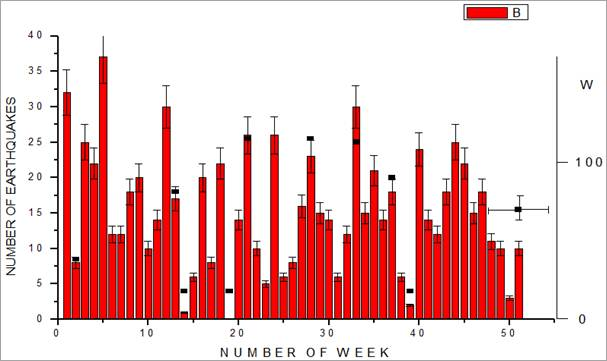Figure 4. The output of solar activity in Wolf numbers W at dates FSA table. 1 (squares) and on average go for the 1730 strongest earthquakes of M> 6.6 minus geo-background19 /week.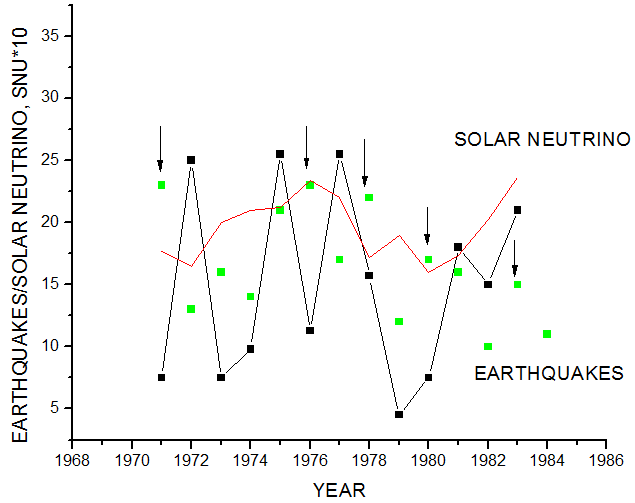Figure 5. The average annual flux of solar neutrinos the Sun Neutrino Units (SNU) at a scale of 10:1 (solid curve). Shows the smoothed curve by 5 points. The point is the number of the strongest earthquakes per year.

In Fig. 5 shows the dependence of the average annual flow of solar neutrinos (, Fig. 5.22, p. 186).The average value of the neutrino flow is 2 solar neutrino units that 3 times smaller than predicted by the standard solar model. Squares points shows the progress of the strongest earthquakes on the A. A. Shpital′naya database, GAO RAS, between frontiers of solar activity 1978 and 1986 years. You can see that the earthquakes repeat the neutrino oscillation. Neutrino minimum 1979 year - precise measurement errors chlorine detector on the release speed of argon -37. This shows the sensitivity of the release of neutrinos by the time the center of mass of the solar system (CMSS) outside of the solar disk on the frontier of the solar activity. Just as dramatically in 1979 year falls seismic activity.

The most interesting  possible explanation of neutrino flow anticorrelation with solar activity, confirmed the same detector in 1990 year , was suggested that M. B. Voloshin, M. I. Vysotsky and L. B. Okhun (Russian Nuclear Physics, 1986, vol. 44, pp. 440, 544, 546).According to their hypothesis, the electronic neutrino with magnetic moment 10-11 Bohr’s magneton as a result of the influence of the solar magnetic field becomes sterile and does not interact with the nucleus of chlorine. Maximum sterilization neutrino occurs during peak solar activity (1978 year– S. A. G.) when the magnetic field of the Sun is the greatest.

6. Time Variation of Solar Neutrino Flux in Chlorine-Argon Experiment 

Constant component Jν = 2.56 SNU = 0.51 37Ar atom/day will be regarded as a constant background which will be deducted from the signal detector to determine the unknown factor of temporal variations of the flux of neutrinos. In our analysis of such deductions will be made for each individual measurement cycle. The results of these operations will be presented in the form of pie charts in a sequential scan of the coordinate α of the Earth the second equatorial coordinate system, the recalculated according to the date of the middle period, each individual cycle measurements. Angle 35° sector and more defined the temporary duration of the measurement. Value of the radius of the sector-its length is equal to the measured magnitude of neutrino flow minus constant component 0.51 37Ar atom/day.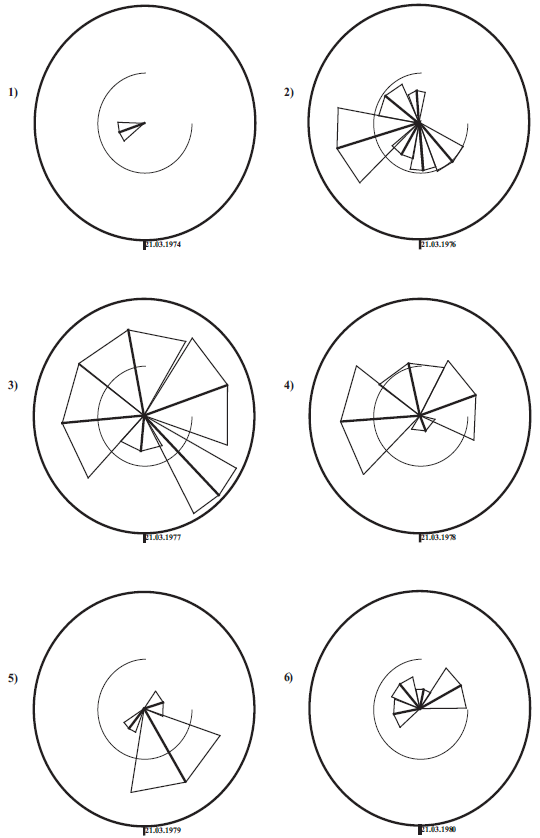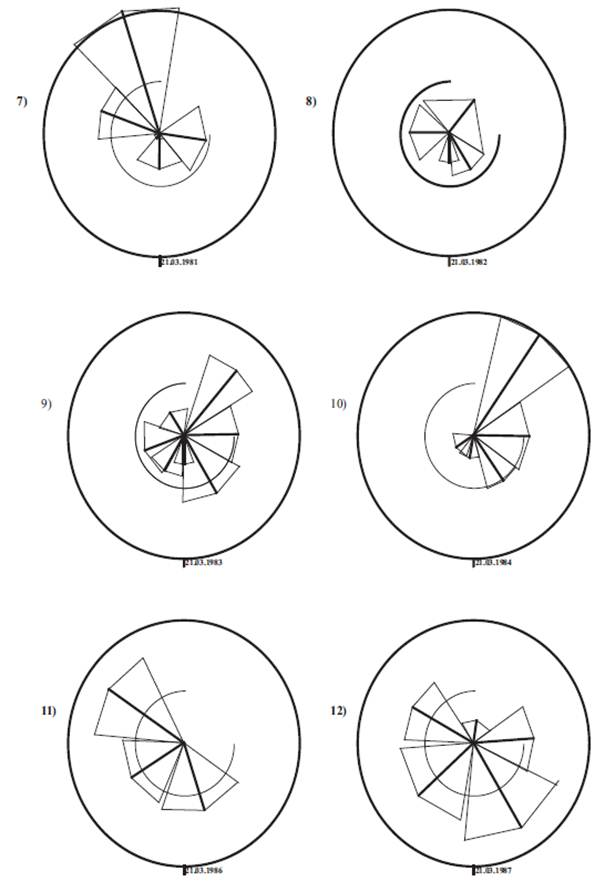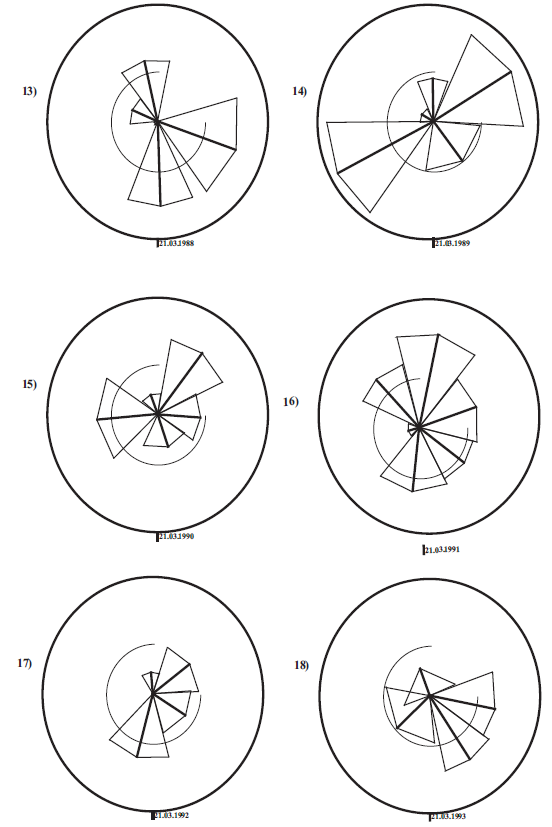Figure 6. Pie charts flow variations of solar neutrinos for the period 1990-1994 years. Constant component of 0.51 37Ar Atom/day marked by an open circle.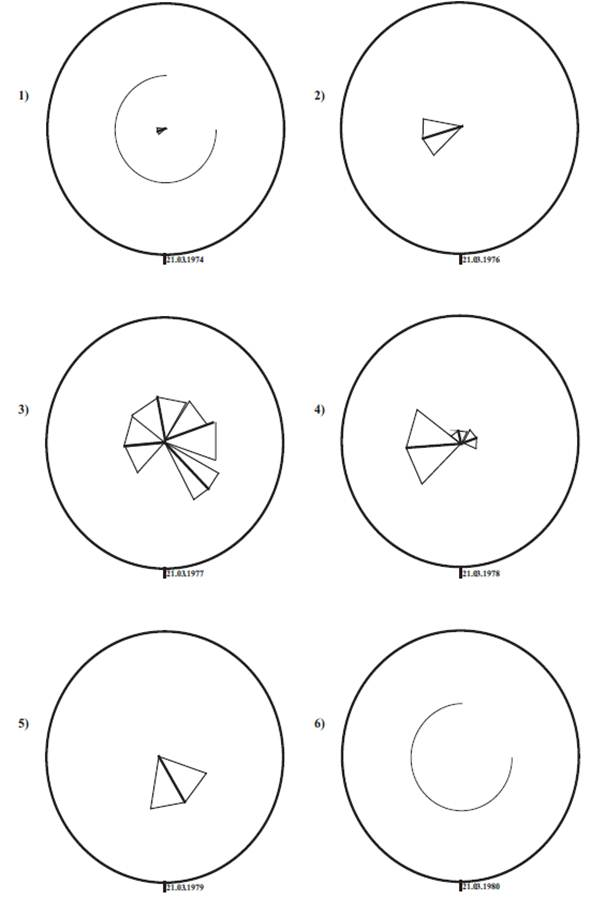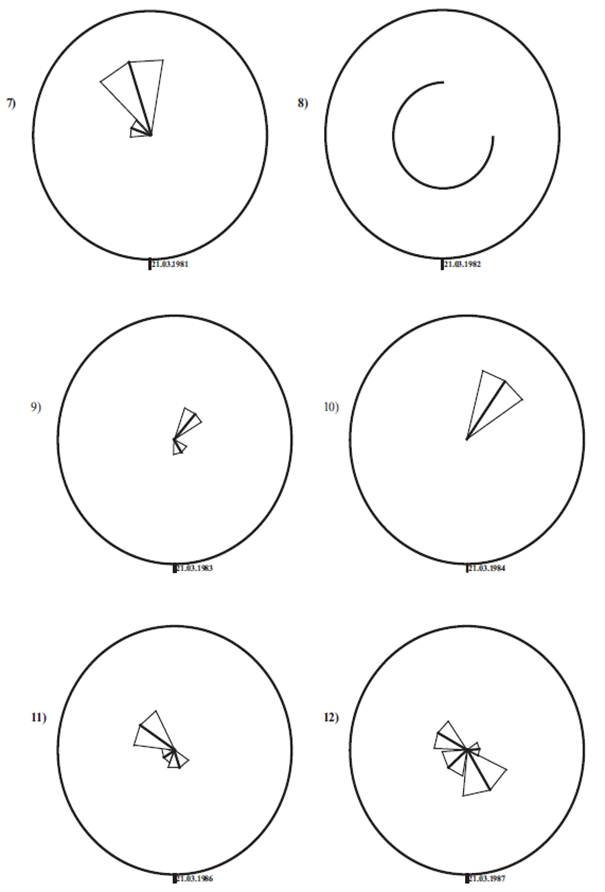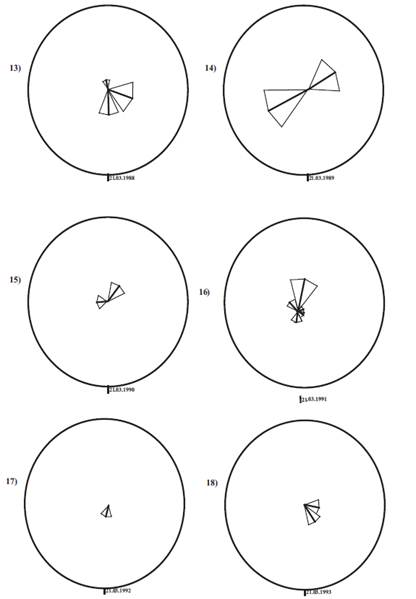Figure 7. Pie charts of solar neutrino flux variations during the period 1990-1994 years, minus the constant component 0.51 37Ar atom/day (open circle).

It should b noted that although and notes the quasi two-year cycle of variations of the solar neutrino flux , we will not produce averaging of biennium, won't do it is because of the informative sharp variations. Separately for each characteristic angle α (310°, 271°, 210° and ± 20°) estimated average value of scan period measurements. It turned out that just such sectors 11-13. The average period of solar neutrino flux measurements amounted to 73° ± 6°, where the value of 6°, scatter to the boundaries of the intervals specified split duration 33, 45, 60 days, etc. small enough to consider all angles except pie the biggest order 100 º (but all these great angles are also included in the review).

The resulting 14 pie charts (from 18) see fig. 6 and 7 are characterized by sharp anisotropy for 4 selected sector directions (centered sector); 4 sector chart after deduction of the constant component of the Chlorine-Argon experiment attributed to noise on the anisotropy. 2 of these 4 are far from FSA 1978, 1986 and 1994.Hence, outside dates FSA therearethe predominantly an isotropic neutrino flux and the whole effect of anisotropy from the remaining 14 charts focus on the dates of the FSA. This conclusion is reinforced by the similar diagram anisotropy strongest earthquakes 1990-2015, with also local maxima distribution release of seismic energy Fig. 3 on the average annual number of earthquakes, exactly localized on dates Frontiers of solar activity.

Time variation of the flow of solar neutrinos is not covered in the standard solar model. On the other hand here, we can see that these are the same effects of spatial anisotropy, characterized by the same angle α = 271° identified on Galactic Gamma-radiation [6-7], on the chart ground experiment  of anisotropy detection cosmological vector AG, the same effect which is described in the model of a quantum oscillator on the string  within the extended standard model, taking into account the gravity with a nonzero torsion. Thus, the data flow time variation of solar neutrinos could well be included in the synthesis of the standard Solar model. You can take it that the selected axis of anisotropy in accordance with [6-7] set on the isotropy of space and time.

7. Time Variation of the Flow of Solar Neutrino Experiment SAGE 

A permanent component of the neutrino flow 81.4 SNU will consider as a constant background, which will be deducted from the signal detector to determine the unknown factor of temporal variations of the flux of neutrinos. In our analysis of such deductions will be made for each individual measurement cycle. The results of these operations will be presented in the form of pie charts in a sequential scan of the coordinate α of the Earth the second equatorial coordinate system, the recalculated according to the date of the middle period, each individual cycle measurements. Angle 28° sector and more defined the temporary duration of the measurement. Value of the radius of the sector-its length is equal to the measured magnitude of neutrino flow minus constant component 81.4 SNU. It should be noted that although and notes the quasi two-year cycle of variations of the solar neutrino flux , we will not produce averaging of biennium, won't do it is because of the informative sharp variations.

The resulting pie charts (from 18) see fig. 8 and 9 are characterized by sharp anisotropy for 4 selected sector directions (centered sector). Just as in chlorine-argon experiment outside dates FSA there are the predominantly an isotropic neutrino flux and the whole effect of anisotropy focus on the dates of the FSA. This conclusion is reinforced by the similar diagram anisotropy strongest earthquakes 1990-2015, with also local maxima distribution release of seismic energy Fig. 3 on the average annual number of earthquakes, exactly localized on dates Frontiers of solar activity.

Figure 8. Pie charts flow variations of solar neutrino experiment SAGE for the period 1990-2005 years minus constant component 81.4 SNU (open circle).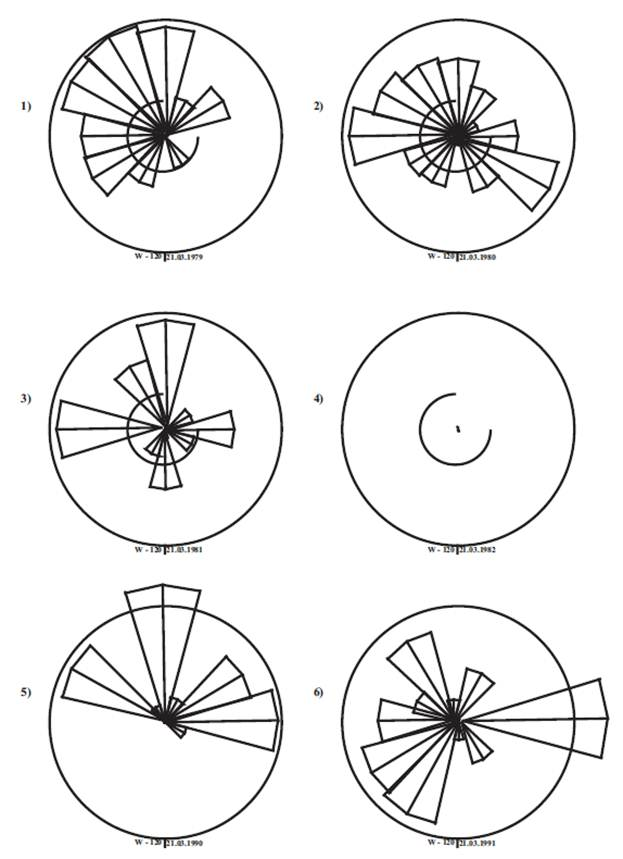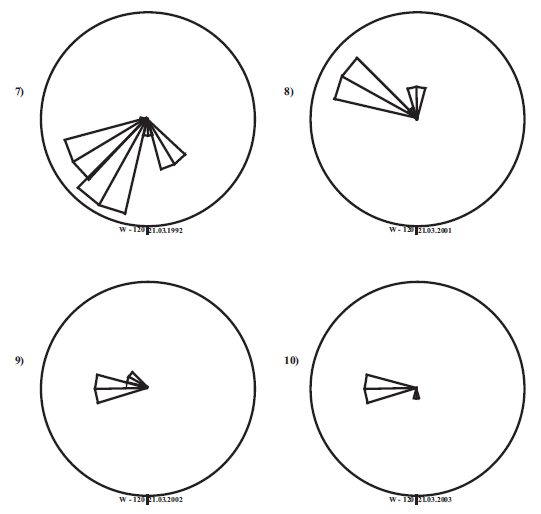Figure 9. Pie charts the variations in solar activity for the period 1979-2003 years.

For comparison the fig. 9 pie charts are temporal variations in solar activity  by number Wolf W during the period 1970-2005 years.

8. Discussion of Results

As a result of processing rice pie charts in Fig. 7, measuring in Chlorine-Argon Homestake experiment  and time variation of the solar neutrino flux were distributed on the following directions:

series A of 12 diagram units-

αA = 271º ± 8º,δ = 23º;

series B of 16 diagram units-

αB = 310º ± 22º,δ = 23º;

series C of 5 diagram units-

ΑC = 208º ± 7º,δ = 23º;

series D of 7 diagram units-

ΑD = 21º ± 23º, δ = 23º.

All these areas are within the dispersion by the time of the cycle experiments 17°-48°.

Pie directions of temporary variations of solar neutrino flux, dedicated to Fig. 8 in SAGE experiment :

series A

αA = 273º ± 12º, δ = 23º;

series B

αB = 312º ± 15º, δ = 23º;

series C

ΑC = 210º ± 0º, δ = 23º;

series E

ΑE = 232º ± 7.5º, δ = 23º.

All these areas are also within the dispersion by the time of the cycle experiments 15º. In addition, you can take it confirmed overlapping the main directions of anisotropy of 271° and 310° in limits permissible under 2σ.

Pie charts of temporal variations of the solar neutrino flux and the seismic energy of the Earth in Fig. 3 discover the apparent similarity in periods of FSA 1978 the EARTH and Neutrino 1977 Fig. 7; 1979 the EARTH and 1985-1986 the EARTH and 1986 Fig. 7; 1993 the EARTH and 1994 Fig. 8; 1995 the EARTH and 1995 Fig. 8; 1996 the EARTH and the 1996-97 Fig. 8; 1999 the EARTH and 1999 Fig. 8; 2000 the EARTH and 2000 Fig. 8, etc. Interestingly, in some cases, the neutrino pie chart repeats the pie chart release seismic energy of the Earth only through 1 year, and a year earlier-you can only see the beginning in neutrino 2-year cycle. It cannot serve as a justification for those solar neutrinos could be the source of earthquakes. Maybe that own time of neutrinos on strongly Non equilibrium closed torsion string, moving at a speed very little-10-13  (relativistic factor 1013) is different from the speed of light in vacuum, in the strongest grade of slowly- in 1013so, the entire process of generating neutrinos for 1 microseconds, the entire process of generating neutrinos for 1 microsecond in the laboratory coordinate system (on Earth) will last exactly 1 year. All of these processes in the unity of the solar-terrestrial relationship  are taking place simultaneously.

In the second Equatorial coordinate system the plane of the ecliptic, to which the orbital velocity vector belongs everywhere land, has an angle δ = 23, º 5. Declination δ radiation from the Sun during the year varies from 23, º 5 in late December to -23, º 5 in late June, taking the value zero during the vernal and autumnal Equinox. Summary on the decline the axes of anisotropy of flux of solar neutrinos defined in section 7, are listed below in the table 2.

Table 2. Coordinates α, δ the axes of anisotropy of temporal variations solar neutrino flow minus the constant component 2.57 SNU in Davis 1970-1994 defined in section 7, in the second Equatorial coordinate system in comparison with coordinates of spatial earthquakes anisotropy axes over the 1990-2015 on chart , Fig. 3 and ground experiment .

 No Solar neutrinos, 1970-1994 Ground experiment  Earthquake 1990-2015 1 α δ α δ α 271º ± 8º 23,º5 293º ± 20º 36º ± 10º 250º-263º 278º-293º Early experiments 283º 26º 293º 36º 303º 46º 2 310º ± 22º < 23º 310º ± 20º 40 307º -322º 3 21º ± 23º ~0º 0º -20º 0º -15º 4 210º ± 7º 0º 200º ± 10º 210º -225º 5 232º ± 8º 12º 230º -240º 225º -240º 6 162º ± 25º -12º 170º -190º 150º -165º

9. Correlation of Solar Cosmic Rays from Seismicity of the Earth

Similar data for solar variations may be obtained by variations in dose RAD detector, measured inside the spacecraft during flight to Mars .RAD started working 9.12.2011-14.07.2012.The measured dose averaged 458-461 μGy/day. Dose from solar Proton events-23-25.01, 7-17.03 at 65-70 day flight and 140-e 17.05.2012. are equal respectively to 4, 19.5 and 1.2 mSv, total 25 mSv. This is 5% of the total dose of 466 mSv. In  calculated the coefficient of anticorrelation between doses with a solar neutron monitor potential modulation control feature A + B/Dl2 in Volts (D1-the number of counts per minute, A and B are constants): 0.77-0.80.

In  was presented a series of reconstructed monthly value of the modulation potential for the period from 1936 through December 2009. The modulation potential parameterizes the energy spectrum of Galactic Cosmic Rays (GCR) near Earth with accuracy sufficient for practical application. The presented series is a composite of three parts. The most reliable reconstruction, which based on data from the world network of neutron monitors, covers the period since 1964 and is characterized by the mean 68% significance level uncertainties of 26 mV. Reconstruction for the period between 1951 and 1964 is based on a few mountain neutron monitors of other type. It is characterized by large uncertainties (formal error is 44 mV). Reconstruction before 1951 is based on ionization chambers. It is characterized by large uncertainties of about 140 mV. The comparison with date of measurements of flux of CR in the stratosphere since 1957 shows agreement since 1964, within 10% in the overall level and with only a few discrepancies which fall upon period of enhanced rate of solar activity minima.

In  according to the year 2012 made recalculation of the average annual solar modulation potential on the neutron monitor up to the year 2020, and on it, using the well-known linear relationship with registered radiation dose, it is obtained that the peak dose mSv/day accounted for at the Frontier of Solar Activity (FSA) date 2002 ± 2.7, as well as on table 3 and the fig. 10 the peaks of the dose for all other FSA dates 1994, 1986, 1978, 1955, 1946 and 1940 without exception. All this peaks correspond to modulation potential minima .

Figure 10. Annual average of reconstructed modulation potential Φ since 1937 (gray line and dots with units on the left axis) and estimated annual dose equivalent rate derived from different models (with units on the right axis) (, Fig. 4). Black, yellow, and blue dots represents the data within the range of modulation Φ between 550 and 810 MV which the RAD cruise measurements covered . Arrows show the year of Frontiers of the solar activity.

Such as in according to (, table 3) only at FSA 1940 was exact minimum of the modulation potential and since 1940 there are not FSA years when was exact minimum of the modulation potential. Of course, years of exact minima of the modulation potential were within the 2.7 years interval near FSA years. So that in whole with above caveats we have amazing match between FSA years of the most numbers of strongest earthquakes per year on fig. 3, 5, when there we stated maximum of the solar neutrino production, in another side and years of the annual average dose maxima in transit to Mars on table 3.

Table 3. The annual average dose in transit to Mars calculated on the annual average of reconstructed solar potential neutron monitor (, fig. 4, p.4).

 Year Dose, mSv/day Year of the Frontier of Solar Activity (FSA) 1940 3.2 maximum 1940 1943 3.4 maximum 1946 4.4 maximum 1946 1948 2 1955 2.5 maximum 1955 1962 1.5 1962 1964 2.5 maximum 1978 2.4 maximum 1978 1986 2.5 maximum 1986 1995 2.2 maximum 1994 2002 2 2002 2004 2.7 maximum 2018 2.5 maximum 2018

This match with dose maximum take place and for the FSA 2018 year. However, it is difficult to say how much dose extrapolation  is justified in this case. Whereas the date of the FSA calculated per thousand years .

10. Conclusion

1.   There is a difference between 1978 year solar maximum on output of neutrinos from other solar maximums.

2.   Difference of point 1 occurs at the moment of coincidence of the year solar maximum with the year of Frontier of solar activity (FSA).

3.   From points 1-2, it follows that the solar neutrino yield significantly associated with FSA dates.

4.   In whole with above caveats we have amazing match between FSA years of the most numbers of strongest earthquakes per year on fig. 3 and 5, when there we stated maximum of the solar neutrino production, in another side and years of the annual average dose maxima in transit to Mars on table 3.

5.   Date of the FSA is determined by the Sun eccentric planets.

6.   Proportionality of solar activity to the exit seismic energy of the Earth on the average annual number of strongest earthquakes in oscillatory response model of dense solar plasma by date all FSA before year 2000 coincided on 7% spread with the schedule release linear dependence of depth seismic energy  from the solar neutrino flux temporal variations on a continuous series of chlorine-argon experiment in 1970 to 1974.

Mass of the muon and tau-neutrinos are calculated. Set the integer 2:5 ratio between the mass of muon neutrinos and muon ultra-light weight torsion. Found that the calculated mass of the tau-neutrino is the same as the largest with heat 3/2 T relic neutrinos. In the standard model T = 2 K. Considering the temperature of the relic neutrinos as the effective gravitational temperature TΓ = 2/9 M for some masses M, it is followed ratio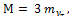corresponding to reactions of the dissolution of the relic neutrinos by three sterile active Tau-neutrinos. A life time of sterile neutrinos such a mass is not less than the life time of the proton. On the other hand this value on the numerical score of the upper limit of the mass of the tau neutrino 10 MeV reaches 13 billion years.

Solar neutrino flux measurements data and its temporal variations in the Chlorine-Argon experiment for the period 1970-1994, examined with particular attention near dated frontiers of solar activity (FSA), which defined by means of the key criterion of 2ln2 in point a significant manifestation of the influence the Sun eccentric planets. Review shall be carried out within the framework of the standard model, taking into account the gravity. The results are presented in the form of pie charts in a consecutive temporary scan on a direct ascent of Earth's orbital position α, recalculated according to the date of the middle of each of the series of measurements. The corners of the sectors 35° and more defined for temporary length of each dimension. The amplitude of the sector is equal to the measured magnitude of neutrino flow minus the constant component 2.57 SNU in chlorine-argon experiment and 81.4 SNU in experiment SAGE. All charts show the priority direction of the solar neutrino flux detector checked by angle α = 271°, δ = 23º (40º -15º) near axis of anisotropy of Galactic Gamma-radiation, as well as a number of less significant in transverse to the axis of the direction within the spread over time of cycle 15° -58° experiments. Researched the relationship between solar activity, seismic activity of the Earth and neutrinos flows registered in chlorine-argon experiment for the period 1970-1994, as well as in the SAGE for the period 1970-2008 years. There is a correlation of solar activity with seismic activity of the Earth and the positive correlation between seismic activity of the Earth and the neutrino flow variations, reaching 95% when taking into account the peculiarities of the matching of dates of solar maximums with FSA dates 1978, 1993-5 and 2002. It turned out that all known and perhaps predicted on 2018 year the greatest average annual values of the flow of solar cosmic rays fall exactly on the years of FSA defined on the motion of the Sun around the center of mass of the solar system because of the influence of the planets eccentric.

References

1. BOEHM F., VOGEL P., Physics of massive neutrinos (Cambridge University Press, Cambridge, 1987).
2. CLEVELAND Bruce T., DAILY T., DAVIS R. etc., Measurement of the solar electron neutrino flux with the Homestake chlorine detector. Astrophysical journal, 496: 505-526, 1998 March 20.
3. The SAGE Collaboration. arXiv: 0901.2200v3 [nucl-ex] 10 Aug 2009.
4. BELOV S. V., SHESTOPALOV I. P., KHARINE. P., On the Interrelations between the Earth Endogenous Activity and Solar and Geomagnetic Activity. DAN [Academy of Science reports]. Vol. 428. No. 1. 104-108 (2009).
5. BAUROV Yu. A., EFIMOV A. A., SHPITALNAYA A. A., Fizicheskaya misl Rossii, No. 1, 1-9 (1997).
6. SYROMYATNIKOVA. G.,LXV International Conference "Nucleus 2015".New horizons in nuclear physics, nuclear engineering, femto- and nanotechnologies. Book of abstracts. June 29 – July 3, 2015, Saint-Petersburg, Russia. P. 173.
7. SYROMYATNIKOV A. G., When Anisotropy of Vacuum Set The Space Isotropy.International Journal of High Energy Physics. Special Issue: Breaking of Space Symmetry in the Masses Spectrum Problem. 2015(to be published).
8. GORBUNOV D. S., Sterile neutrinos and their roles in particles physics and cosmology, Uspekhi Fizicheskikh Nauk 184 (5) 545 ± 554 (2014).
9. PILAFTSISA, UNDERWOOD T. E., J Phys. Rev. D 72 113001 (2005); hep-ph/0506107
10. ASAKA N., SHAPOSHNIKOV M., Phys. Lett. B 620 17 (2005); hep-ph/0505013.
11. SYROMYATNIKOV A. G. On Similarity between All-Known Elementary Particles and Resonances Mass Spectrum and Nuclear Atomic Weight. Univ. J. Phys. and Appl. 2(2)76-79. DOI: 10.13189/ujpa.2014.02023.
12. SYROMYATNIKOV A. G., Comparison Analogy Between Properties of Hypernucleus and Supernucleus with Properties of the Elementary Particles and Resonances Electroproduction by Spin Shock Waves.International Journal of High Energy Physics. Special Issue: Breaking of Space Symmetry in the Masses Spectrum Problem. 2015 (to be published).
13. NAKAMURA K. et al. [Particle Date Groups], J PG 37, 075021 (2010) and 2011.
14. SYROMYATNIKOV A. G., On some feature of possible torsion effects on observables at hadron colliders, Int. J. Geom. Meth. Mod. Phys (2015) DOI:10.1142/S0219887815500802
15. SHESTOPALOV I. P., KUZHEVSKY B. M., KHARIN E. P., Correlations flows neutrinos with seismicity of the Earth. The hypothesis on elocation neutrinos in a period of deep strong earthquakes, Inzhenernaya fizika. No 1. 2014. P. 4-12.
16. SYROMYATNIKOV A. G. Physical effects in Conformal Gauge Theory of Gravitation (LAP Lambert Academic PublishingGmbH & Co. KG, Saarbrucken, Germany, 2012).
17. SYROMYATNIKOV A. G., On connection between a Solar activity and Solar system dynamics. – Works of III International Congress "Weak and Hyperweak FIELDS and RADIATIONS in Biology and Medicine", Saint-Petersburg, 01-04.07.2003, p. 90.
18. SYROMYATNIKOV A. G., ZAKOLDAEV Yu. A., Depth distribution of rocks under the Geospace Universal X-structure of the Earth crust and upper mantle. (Petropolis, Saint-Petersburg, 2011).
19. Web-site: http://www.ceme.gsras.ru.
20. VASILIEVA G. F., NESTEROV M. M., CHERNIKCH Yu. V., The generation process in the Sun's magnetic field when you change the dynamic parameters of the solar system, A series "Problems of research of the Universe". Issue 25. Part II. Saint-Petersburg, Russia, 2002). P. 303-320.
21. BAKAL Dzh., Nejtrinnaja astrofizika [Neutrino astrophysics]. Transl. from English. M.: Mir [Moscow: Publishing House "Peace"], 1993. 624 p.
22. BAUROV Yu. A., J. Mod. Phys. Vol. 3. 2012. P. 1744.
23. GUO G., ZEITLIN C., WIMMER-SCHWEINGRUBER R. F. et al.: Variations of dose observed by MSL/RAD in transit to Mars: arXiv: 1503.0663v1 [physics.space-ph] 23 May 2015.
24. ISOSKIN I. G., BAZILEVSKAYAG. A., and KOVALTSOV G. A. (2011), Solar modulation parameter for cosmic rays since 1936 reconstructed from ground-based neutron monitors and ionization chambers, J. Geophys. Res., 116, A02104, doi: 10.1029/2010JA016105.

 Contents 1. 2. 3. 4. 5. 6. 7. 8. 9. 10.
Article ToolsAbstractPDF(6660K)